﻿ Magnetic Field Distribution of Coil Antenna with Capacitor for ResonanceExamples | Product | Murata Software Co., Ltd.# Example11Magnetic Field Distribution of Coil Antenna with Capacitor for Resonance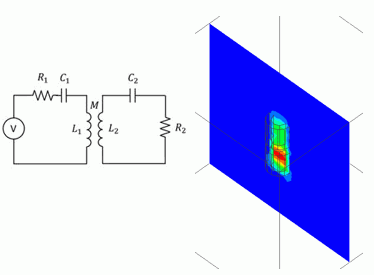General

• Magnetic field distribution at the LF band (around 130KHz) is analyzed.

• The magnetic flux density distribution, the circuit current, and the inductance are calculated.

• The analysis is coupled with the external circuit.
Resonant capacitors are placed in the external circuit.

• Unless specified in the list below, the default conditions will be applied.

### Analysis Space

 Item Setting Analysis Space 3D Model Unit mm

### Show Results

 Item Setting Solver Magnetic Analysis [Luvens] Analysis Type Transient Analysis Options Select [Inductance Calculation].

The Transient Analysis tab is set up as follows.

50KHz AC is input. To reproduce its waveform well, the timestep is set by dividing the periodic time of 50KHz by 12.

Tab

Setting Item

Setting

Transient Analysis

Timestep

Automatic

Table

 Number Calculation steps Output steps Timestep [s] 1 12*4 1 1.0/(12*130e3)

External circuit is as follows.

An AC power is placed on the primary side.

The primary coil and the secondary coil are connected on the model side.

The capacitors are connected to both of the primary and secondary coils in order to have the LC series resonance.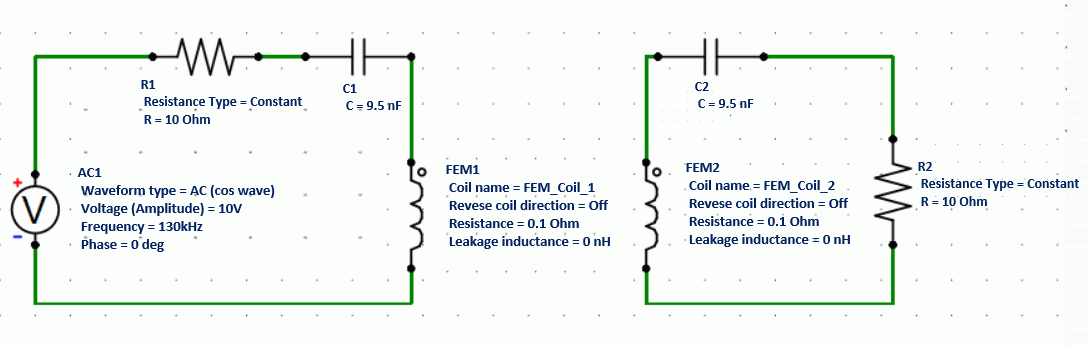### Model

The primary coil (Coil1) and the secondary coil (Coil2) are defined by the loop coil.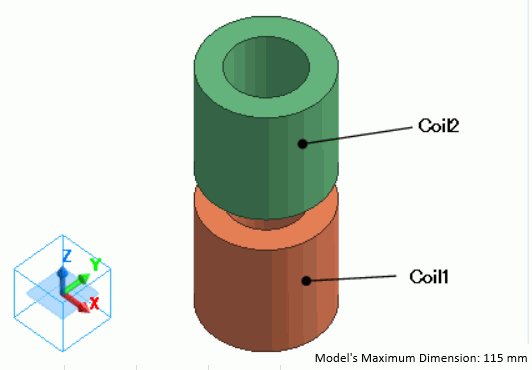### Body Attributes and Materials

 Body Number/Type Body Attribute Name Material Name 5/Solid Coil1 008_Cu * 4/Solid Coil2 008_Cu *

* Available from the material DB

The body attribute is set up as follows.

Each coil is linked to the FEM coil on the external circuit.

 Body Attribute Name Tab Setting Coil1 Current Waveform: External circuit coupling Coil name on the circuit: FEM_Coil_1 Turns: 100[Turns] Direction: Loop Coil/Magnetic Field Direction Direction Vector of Magnetic Field: X=0, Y=0, Z=1 Coil2 Current Waveform: External circuit coupling Coil name on the circuit: FEM_Coil_2 Turns: 100[Turns] Direction: Loop Coil/Magnetic Field Direction Direction Vector of Magnetic Field: X=0, Y=0, Z=1

No setting.

### Results

The distribution of the magnetic flux density at 0.25641e-5[s] is shown below.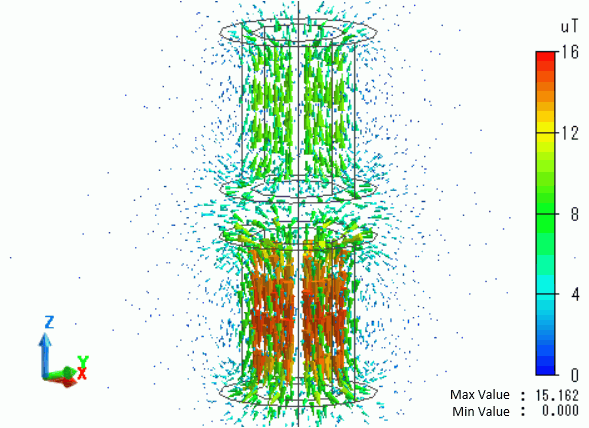The circuit current waveforms are as follows.

The current of the primary coil (blue wave) is a sine wave and shifted from the voltage power (cosine wave) by π/2 phase.

The currents of the primary coil and the secondary coil show the opposite phases.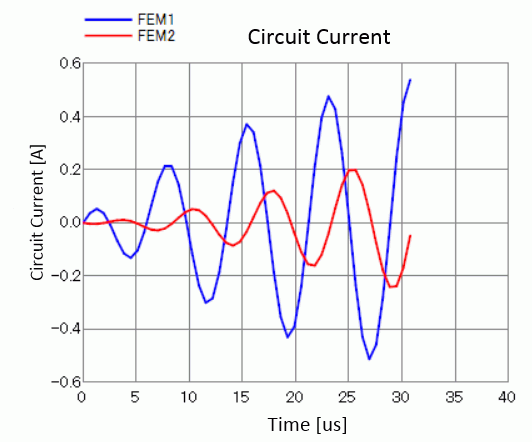The result of inductance calculation is shown below.

Self inductance [H] Mutual inductance [H]

﻿